# FPGA Implementation of Fixed-Point Model for a Single-Phase AC-DC Converter with Unity Power Factor

FPGA Implementation of Fixed-Point Model for a Single-Phase AC-DC Converter with Unity Power Factor
Department of Electronic, Faculty of Technology, University Mohamed Boudiaf, M’Sila 28000, Algeria

LAS Laboratory, Electrical Engineering Department, Faculty of Technology, Ferhat Abbas University, Setif 1 19000, Algeria

Corresponding Author Email:
Page:
181-187
|
DOI:
https://doi.org/10.18280/jesa.550204
22 March 2022
|
Accepted:
19 April 2022
|
Published:
30 April 2022
| Citation

OPEN ACCESS

Abstract:

This paper presents a design of digital control based on a fixed-point model for an application of a single phase AC-DC boost Converter with a high power factor and low current distortion. The system uses a simple closed-loop control with a slow voltage loop (outer loop) to stabilize the output DC bus voltage at the required level and a fast current loop (inner loop) to achieve input power factor correction. The digital control uses a fixed-point structure that improves the control accuracy without increasing the number of arithmetic operations and does not require specific running conditions. The behavior of the system is fully verified by using a digital simulation under Matlab/Simulink environment and experimentally with an implementation in real-time using Xilinx ISE 14.7 software, hardware description language VHDL, Xilinx FPGA ML605 board, and EVAL-AD7656 evaluation board for high-speed analog-to-digital conversion. The obtained results show a good agreement between experiments and simulations for steady-state and transient-responses.

Keywords:

fixed-point, FPGA, power factor corrector, hysteresis current controller, proportional-integral controller

1. Introduction

Recently, due to the advancement in digital electronics, the field programmable gate arrays (FPGAs) have appeared as new solutions for the implementation of complex control algorithms. Compared to the conventional implementation solutions (processor and microcontroller chips) , the FPGAs are fully programmable, the updates and the adaptations can be carried out even after delivery to the customer. The development cycle of complex systems is shortened by the early availability of product prototypes (intellectual property IP cores), and the developed hardware can be updated in the field, resulting in an extremely short time-to-market in FPGAs, the complex tasks can be solved by software implementations; also the executions can be obtained with a significant speed via the tasks parallelization [2-5].

It should be noted that to reduce cost and time, the FPGAs enable to test the developed algorithms even before conception. This technique is called FPGA in the loop (FIL) where the system tuning and its validation can be obtained easily and safely. The FIL combines the software flexibility and real-time accuracy with a hardware execution speed [6, 7]. The controlled system (generators, sensors, observers and mechanical actuators…etc.) can be simulated on a host computer PC with a software environment such as Matlab-Simulink and at the same time, the control algorithm can be executed in real-time on the hardware (FPGA) .

In some previous research works, several digital controllers using FPGAs were realized for the single-phase AC-DC boost power factor corrector (PFC) topology. The algorithms of these controllers are based on different ideas and analysis [9-15].

In this context, the work of this paper deals with an application of a non-polluting single-phase AC-DC converter digitally controlled by the ML605 Xilinx FPGA board . The topology is composed of a single phase diode-bridge associated with a DC/DC boost converter [17, 18]. The main goal of its closed-loop control system is to maintain the DC bus voltage at the required level and ensure the unity power factor correction (the input current drawn from the source should be sinusoidal and in phase with the voltage).

A digital simulation using Matlab/Simulink of both the power and control systems is performed and the digital controller is developed and implemented in real-time on FPGA with several tests. The control algorithm is implemented using 16-bit fixed-point operator. The use of fixed-point arithmetic operators offers significantly less area, a shorter latency, and less power consumption [5, 19, 20]. The selection of the data range of a suitable fixed-point representation format for FPGA implementation is obtained from the Matlab/Simulink model-based design. The illustrated results confirm the good agreement between the simulation and the experimental study.

The remaining of this paper is organized as follows. The conventional single-phase AC-DC converter is presented in Section 2. Section 3 presents the non-polluting single-phase AC-DC converter and its closed-loop control system. The implementation of the control algorithm in FPGA is presented in Section 4. Section 5 describes the internal control module developed in FPGA. The obtained simulation results for different operating conditions are illustrated and discussed in Section 6. Section 7 presents the experimental implementation on the FPGA system board and the obtained experimental results. Some conclusions are given in Section 8.

2. Conventional Single Phase AC-DC Converter

Semiconductor diodes are generally used in rectifier bridges, which rectify an alternating current (AC) to direct current (DC). They can produce only a rectified DC voltage of a fixed average value. The conventional single-phase AC-DC converter contains generally of a full-bridge rectifier followed by a capacitor filter to reduce the ripples present in the output voltage (Figure 1) [18, 21]. The input current of such a rectifier circuit comprises current pulses that result a high harmonic distortion of the input current. In this topology, the diodes conduct only during the charging of the capacitor; when the instantaneous source voltage is greater than the capacitor voltage (for short periods of time). The typical total harmonic distortion of input current for this type of rectifier is generally in the range of 55% to 65% and the power factor is about 0.6 . Figure 2 shows the typical simulated line voltage, capacitor voltage, and line current waveforms.

## 1.png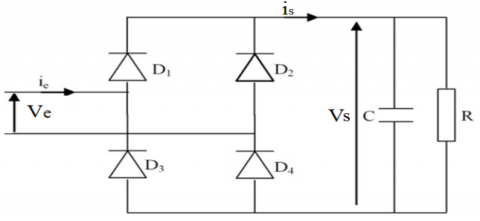Figure 1. Single-phase full-bridge rectifier followed by a capacitor filter

## 2.png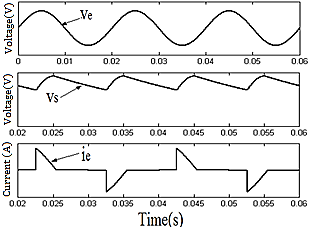Figure 2. Typical simulated AC source voltage, capacitor voltage and input current waveforms

3. Non-Polluting Single Phase AC-DC Converter

While the capacitor filter used in the conventional rectifier significantly eliminates the ripple from the output DC voltage and introduces high distortions to the input current (current discontinuously with short pulses). Several passive and active methods were used to correct the power factor of this converter and make the total harmonic distortion (THD) of its input current at the required level . The limits of the harmonic pollution are imposed typically by the standard norm IEC 61000-3-2 which evaluates and fixes the limit for equipment that draws input current less than or equal to 16A per phase [18, 21, 23].

The power factor depends on both current harmonic content and displacement power factor. The structure depicted in Figure 3 generally acts as a pre-regulator connected to an AC source to bring its power factor close to unity. The single-phase source provides its energy to resistive load via a PFC stage composed of a single phase diode-bridge associated with a DC/DC boost converter. This topology is characterized by high efficiency; it offers a good DC bus voltage regulation and a unity power factor at the AC source side with a closed-loop control.

The control circuit illustrated in Figure 3 is performed with a slow voltage loop (outer loop) to stabilize the DC bus voltage and a fast current loop (inner loop) to achieve a near unity power factor. For the first loop, a proportional-integral (PI) controller is used to regulate the DC bus voltage. Its output (magnitude of the reference source current) is multiplied by a rectified sinusoidal signal synchronized with the source voltage. The obtained result is considered as the instantaneous reference inductor current iLref. For the second loop, the inductor current iL is forced to track its reference iLref by a hysteresis current controller within the desired bandwidth. This regulator is characterized by simplicity and high robustness.

## 3.png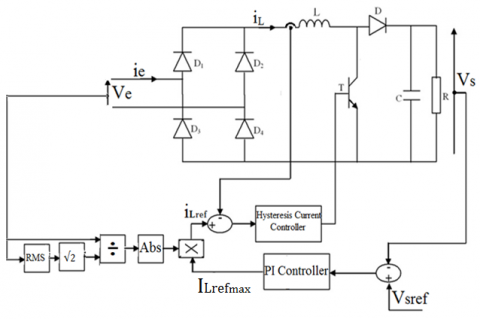Figure 3. AC-DC boost PFC with current and voltage loop control

The DC bus voltage Vs is sensed and compared with a reference value Vsref. The obtained error is used as input for the PI controller and the output of the PI controller is ILrefmax.

Assuming that $i_{L} \cong i_{L r e f}$ and considering that the converter components are ideal, with this assumption a power balance is independent of the presence of the converter and the expressions of the instantaneous powers can be equal on the source and the load sides as:

$\frac{V_{\max } \cdot I_{\max }}{2} \cong V_{S}\left(C . \frac{d V_{S}}{d t}+\frac{V_{S}}{R}\right)$               (1)

with:

Vmax: Magnitude of the source voltage;

Imax: Magnitude of source current.

(1) can be rewritten as:

$\frac{V_{s}^{2}}{R}+\frac{C}{2} \cdot \frac{d V_{s}^{2}}{d t}=\frac{V_{\max } I_{\max }}{2}$              (2)

with:

$V_{s}=\bar{V}_{s}+\tilde{V}_{s}$

$I_{\max }=\bar{I}_{\max }+\tilde{I}_{\max }$

(2) is of a non-linear nature. The linearization around the operating point gives the transfer function of the system:

$\frac{\tilde{V}_{s}}{\tilde{I}_{\max }}=\frac{V_{\max }}{4 \bar{V}_{s}} \cdot \frac{R}{1+\frac{R C}{2} S}$                (3)

The scheme in closed-loop is given by Figure 4.

## 4.png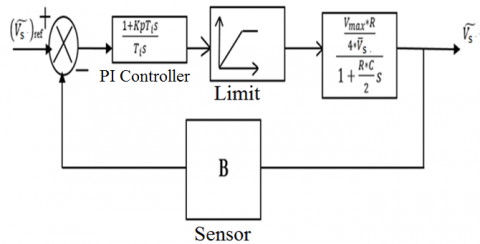Figure 4. PI controller for DC bus voltage regulation

The parameters of PI controller are calculated by dominant-pole compensation method as:

$\left\{\begin{array}{c}K_{p}=\frac{R C}{2} K i \\ K_{i}=\frac{1}{T_{i}}=\frac{8 \pi f_{c} \bar{V}_{s}}{B R V_{\max }}\end{array}\right.$                  (4)

with:

Kp: gain of proportional action.

Ki: gain of integral action.

B: attenuation of the measured output voltage.

fc: the voltage closed-loop crossover frequency.

4. Implementation of Control Algorithm on FPGA

The global control system of the structure presented by Figure 3 is digitally implemented on the ML605 FPGA board, as illustrated in Figure 5. It contains three parts: analog-to-digital converter AD7656 , ML605 FPGA and XM105 mezzanine card . The implemented control program requires two voltage sensors and one Hall Effect current sensor to detect the source voltage, the DC bus voltage, and the inductor current respectively.

## 5.png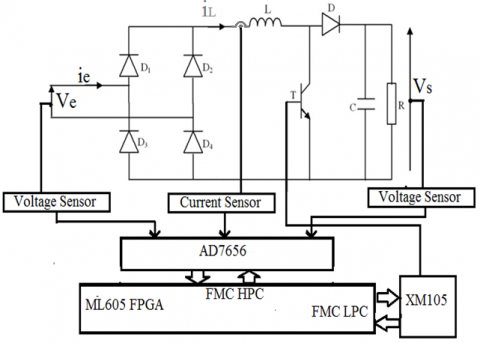Figure 5. Digital control algorithm system for AC-DC boost PFC

The AD7656 is used to perform the analog-to-digital conversion of the captured signals (source voltage, DC bus voltage, and inductor current). The AD7656 has six-channel 16-/14-/12-bit, simultaneous sampling and high-speed parallel and serial interfaces. It can be interfaced with FPGA, microprocessors, or digital signal processors (DSPs). It operates with a throughput going of up to 250 kSPS and contains a low noise, wide bandwidth track-and-hold amplifiers that can handle input frequencies up to 4.5 MHz .

4.2 Ml605 FPGA

The ML605 FPGA controls and processes the AD7656 data acquisition system, and then generates the control signal. The ML605 board enables users to develop designs targeting the Virtex®-6 XC6VLX240T-1FFG1156 FPGA. It permits the application of various research fields based on FPGA such as embedded processing systems and hardware in the loop co-simulation, signal and imaging processing...etc. The ML605 board has one 2.5V LVDS differential 200 MHz oscillator and 66 MHz 2.5V single-ended clock socket provided for user applications. Some commonly used features include a DDR3 SODIMM memory, an 8-lane PCI, Express® interface, a tri-mode Ethernet PHY, general-purpose I/O, and a UART. Additional user- desired features can be added through mezzanine cards attached to the onboard high-speed VITA-57 FPGA Mezzanine Connector (FMC) high pin count (HPC) expansion connector, or the onboard VITA-57 FMC low pin count (LPC) connector .

4.3 XM105 mezzanine card

The XM105 mezzanine card is used to output the generated signal and send it to transistor IGBT via an integrated card that ensures the insulation and the dead time of control signals .

5. FPGA Internal Control Module Design

To design the overall control system, the internal control module is written by using VHDL language with a structural description, where each part of the control system is designed in different levels of abstraction, according to the required accuracy and the complexity of each model.

## 6.png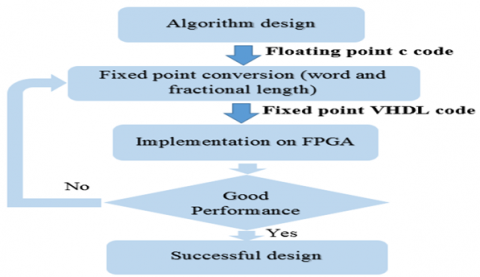Figure 6. Different steps for the design implementation flow of a digital control algorithm

The flowchart of Figure 6 summarizes the different steps used for the implementation of the digital control algorithm.

1. Design and simulation of the control algorithm under Matlab/Simulink software.

2. Determination of suitable fixed-point representation (word and fractional length) using the fixed-point and HDL-Coder tools integrated into Matlab/Simulink Software  or by manual calculation .

3. Implementation of the control algorithm.

4. Verification of the control algorithm performance. If the performance is satisfied, a successful design is approved; else the execution returns to step 2 again.

The AC-DC boost PFC system is controlled by a closed-loop control utilizing a PI controller and a hysteresis current controller as shown in Figure 3.

The output of the PI controller in the discrete-time domain is given by [7, 26]:

$\mathrm{I}_{\text {Lrefmax }}(\mathrm{k})=\mathrm{I}_{\text {Lrefmax }}(\mathrm{k}-1)+\mathrm{T}_{\mathrm{s}} \mathrm{K}_{\mathrm{i}} \mathrm{e}(\mathrm{k})-K_{p} e(k-1)$                (5)

where:

$\mathrm{T}_{\mathrm{s}}$: Sampling period.

$e(k)$ and $e(k-1)$ Are the DC bus voltage errors at k and k-1 respectively.

For the hysteresis current controller, an RS flip-flop followed by a comparator is used. The comparator has an upper threshold (VH) and a lower threshold (VL). The RS flip-flop receives the signals provided by the comparator and generates the control signal of transistor IGBT.

The VHDL behavioral description of the whole system is performed in a 16-bit fixed-point format. Figure 7 shows the 16-bit fixed-point data format of the AC-DC boost PFC control algorithm generated by fixed-point tools in Matlab/Simulink.

## 7.png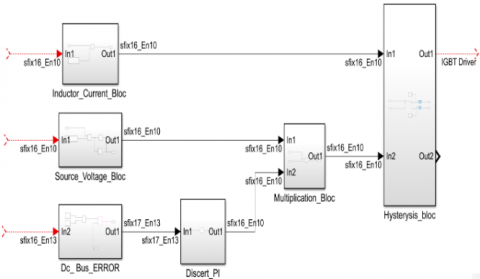Figure 7. 16-bit fixed-point data format Matlab/Simulink model of AC-DC boost PFC control algorithm

## 8.png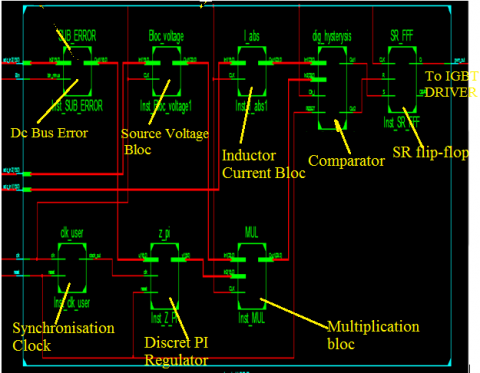Figure 8. Implemented 16-bit fixed-point data format circuit of the AC-DC boost PFC control algorithm

The FPGA hardware implementation model 16-bit fixed-point data format generated by Xilinx ISE software is shown in Figure 8.

The connection between AD7656 and ML605 FPGA should be tested to confirm the correct data and timing requirements. The AD7656 is connected to ML605 FPGA through the FMC HPC connector. The AD7656 operates at a frequency of 100 MHz generated by the ML605 FPGA. The ML605 FPGA sends a start signal acquisition when the BUSY signal is at high level, AD7656 starts sampling, when the sampling is over, the BUSY signal passes in low level, the FPGA reads out the six channels. The whole conversion time takes 4μs. Figure 9 shows the interface signals and data bus between ML605 FPGA and AD7656.

## 9.png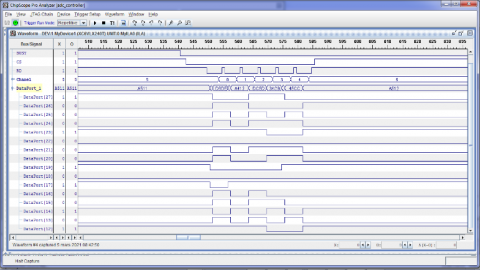Figure 9. Interface signals and data bus between ML605 FPGA and AD7656

6. Simulation Results

In order to verify the feasibility of the AC-DC boost PFC, several simulation tests are performed under Matlab/Simulink environment for different operating conditions. The demonstration is done for a low-power application, where the parameters of the power circuit are given in Table 1. The hysteresis band is fixed to 0.1 A. For the calculation of the PI controller parameters, the crossover frequency is 5 Hz and the attenuation of the measured output voltage is of 0.01.

Table 1. Power circuit parameters

 Parameters values AC Source voltage (RMS) Ve 10 V AC Source frequency f 50 Hz DC bus voltage Vs 20 V DC bus capacitance C 1100 µF DC boost converter inductance L 10 mH Load resistance R 200 Ω

Figure 10 shows the waveforms of source voltage and current for the steady-state operating. Note that the output DC bus voltage is fixed to 20 V. It is well observed that the resistive load is fed by a non-polluting converter, where the source current has a near-sinusoidal waveform and it is perfectly in phase with the source voltage; this can show that the source power factor is very close to unity.

The waveforms of the output DC bus voltage and the source current for the step change of Vsref are presented in Figure 11. After a short transient, the output DC bus voltage is maintained close to its new reference value with a good stability. The source current has a near-sinusoidal waveform.

## 10.png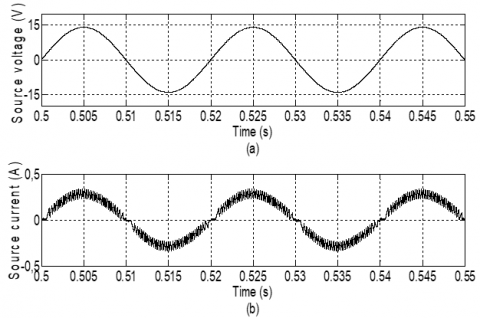Figure 10. Simulation results in steady-state: (a) Source voltage, (b) Source current

## 11.png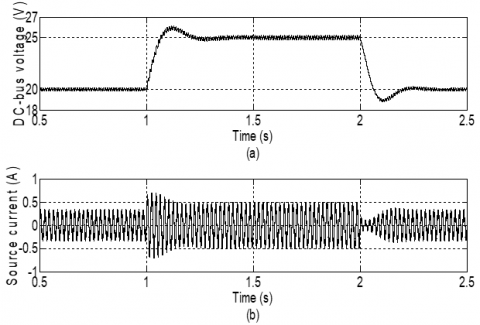Figure 11. Simulation results for the step change of Vsref (increasing from 20 V to 25 V and decreasing from 25 V to 20 V): (a) DC bus voltage, (b) Source current

Figure 12 shows the waveforms of the output DC bus voltage and the source current for the step change of resistive load. It can be observed that after a short transient, the output DC bus voltage is maintained close to its reference value of 20 V. The unity power factor operation is successfully achieved.

## 12.png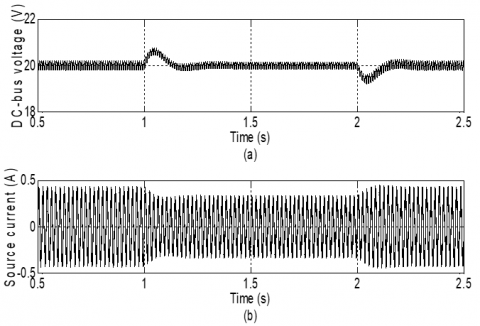Figure 12. Simulation results for the step change of resistive load (increasing from 150 Ω to 200 Ω and decreasing from 200 Ω to 150 Ω): (a) DC bus voltage, (b) Source current

7. Experimental System and Results

Figure 13 shows the experimental test bench prototype performed in laboratory to validate the simulation results and the efficiency of the AC-DC boost PFC system. For the implemented control program, two voltage sensors (with an attenuation of 0.01) and one Hall Effect current sensor (with an attenuation of 0.1) are used to sense the source voltage, the DC bus voltage, and the inductor current respectively. The sensed signals are introduced via a high-speed analog-to-digital converter AD7656. The control program is simulated using Matlab/Simulink and Xilinx ISE 14.7 environments and implemented in real-time through the ML605 Xilinx FPGA. The provided control signal is drawn by the XM105 mezzanine card and is sent to IGBT via the gate drive circuit.

## 13.png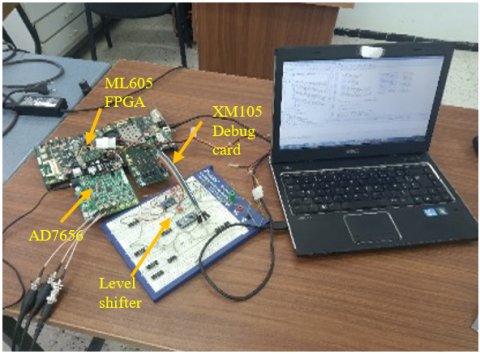Figure 13. Experimental test bench with FPGA system control

For the experimental tests, the same parameters of the power circuit and control data are used as in digital simulation tests.

Table 2 shows the FPGA resources of implemented method. Compared to Table 2 in ref. , this method uses less FPGA resources.

Figure 14 shows the waveforms of the source voltage and current obtained during the operating in steady-state (with 20 V of DC bus voltage). It is well noted that the AC-DC boost PFC draws a sinusoidal current at high quality and in phase with the voltage, which leads to a perfect power factor correction.

Table 2. FPGA resources used by the implemented method

 Number of Block RAMs 0 Number of Slice LUTs 268 Number of fully used FIP-FLOPs 369 Number of DSPs 1

## 14.png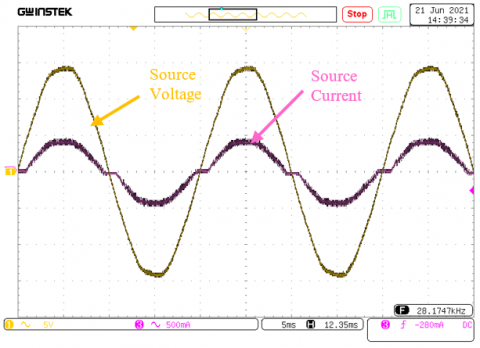Figure 14. Experimental results: Source voltage and current in steady-state

## 15.png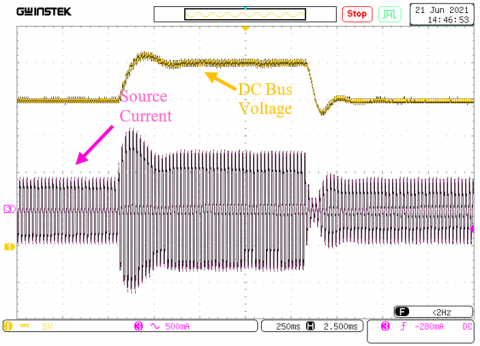Figure 15. Experimental results: DC bus voltage and source current for the step change of Vsref (increasing from 20 V to 25 V and decreasing from 25 V to 20 V)

## 16.png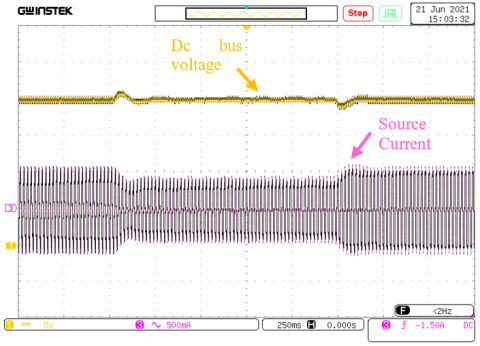Figure 16. Experimental results: DC bus voltage and source current for the step change of resistive load (increasing from 150 Ω to 200 Ω and decreasing from 200 Ω to 150 Ω)

The waveform of the output DC bus voltage and the source current for the step change of Vsref is presented in Figure 15. After a short transient, the output DC bus voltage is well-regulated around to its new reference value.

Figure 16 shows the waveform of output DC bus voltage and the source current for the step change of resistive load. After a short transient, the output DC bus voltage is maintained stable around to its reference value of 20 V.

8. Conclusion

This paper has described a digital control based on a fixed-point model for a non-polluting single phase AC-DC converter.  The latter improves the control accuracy without increasing the number of arithmetic operations and does not require specific running conditions. The converter comprises a diode bridge rectifier followed by a DC/DC boost converter. It is controlled by using a closed-loop control to ensure the correction of the input power factor and the regulation of the output DC bus voltage. The system is simulated under Matlab/Simulink environment and experimentally tested with an implementation in real-time using Xilinx ISE 14.7 software, hardware description language VHDL, Xilinx FPGA ML605 board, and an AD7656 high-speed analog-to-digital converter. The experimental results are in good agreement with the simulation results for different operating conditions.

This application can be extended to renewable energies area, especially for the stand-alone photovoltaic systems, where the investigated topology can be used as an alternative to batteries in order to solve the main drawback of the energy production intermittency; i.e. the photovoltaic system can be interfaced with the single-phase grid (as secondary source) via this topology to ensure a continuous power supply.

References

 Maksimovic, D., Zane, R., Erickson, R. (2004). Impact of digital control in power electronics. In Proc. IEEE Int. Symp. Power Semicond. Devices Ics, pp. 13-22. https://doi.org/10.1109/WCT.2004.240284

 LaMeres, B.J. (2019). Introduction to Logic Circuits & Logic Design with VHDL. Springer Nature Switzerland AG. ISBN: 978-3-319-34194-1.

 Andina, J.J.R., De la Torre Arnanz, E., Peña, M.D.V. (2017). FPGAs: fundamentals, advanced features, and applications in industrial electronics. CRC Press. https://doi.org/10.1109/MIE.2017.2704499

 Sklyarov, V., Skliarova, I., Barkalov, A., Titarenko, L. (2014). Synthesis and optimization of FPGA-based systems. Springer Science & Business Media. https://doi.org/10.1007/978-3-319-04708-9

 Peter, J.A. (2008). Digital design: An Embedded Systems Approach Using VHDL. Morgan Kaufmann Publishers.

 Zamiri, E., Sanchez, A., Yushkova, M., García, M.S.M. (2021). Comparison of different design alternatives for hardware-in-the-loop of power converters. Electronics, 10(8): 926. https://doi.org/10.3390/electronics10080926

 Benhamadouche, A.D., Djahli, F., Ballouti, A., Sahli, A. (2019). FPGA-based hardware-in-the-loop for multi-domain simulation. International Journal of Modeling, Simulation, and Scientific Computing, 10(4): 1950020. https://doi.org/10.1142/S179396231950020X

 MathWorks® Documentation. (2021). HDL Verifier™ User’s Guide. In MathWorks., www.mathworks.com.

 Zhang, W., Liu, Y., Wu, B. (2006). A new duty cycle control strategy for power factor correction and FPGA implementation. IEEE Trans. Power Electronics, 21(6): 1745-1753. https://doi.org/10.1109/TPEL.2006.882922

 Garcia, A., de Castro, A., Garcia, O., Azcondo, F.J. (2009). Pre-calculated duty cycle control implemented in FPGA for power factor correction. In 2009 35th Annual Conference of IEEE Industrial Electronics, pp. 2955-2960. https://doi.org/10.1109/IECON.2009.5415383

 Sanchez, A., de Castro, A., López, V. M., Azcondo, F.J., Garrido, J. (2013). Single ADC digital PFC controller using precalculated duty cycles. IEEE Transactions on Power Electronics, 29(2): 996-1005. https://doi.org/10.1109/TPEL.2013.2256931

 de Castro, A., Zumel, P., García, O., Riesgo, T., Uceda, J. (2003). Concurrent and simple digital controller of an AC/DC converter with power factor correction based on an FPGA. IEEE Transactions on Power Electronics, 18(1): 334-343. https://doi.org/10.1109/TPEL.2002.807106

 Jappe, T.K., Mussa, S.A., Rosendo, R.H.S. (2010). Synchronous state machine inner FPGA controlling PFC boost converter. In 2010 IEEE International Symposium on Industrial Electronics, pp. 1097-1102. https://doi.org/10.1109/ISIE.2010.5636865

 Lamo, P., de Castro, A., Sanchez, A., Ruiz, G.A., Azcondo, F.J., Pigazo, A. (2021). Hardware-in-the-loop and digital control techniques applied to single-phase PFC converters. Electronics, 10(13): 1563. https://doi.org/10.3390/electronics10131563

 Benyamina, A., Moulahoum, S. (2019). FPGA in the loop based single phase power factor correction. In 2019 Progress in Applied Electrical Engineering (PAEE), pp. 1-6. https://doi.org/10.1109/PAEE.2019.8788997

 ML605 Hardware User Guide. http://www.xilinx.com.

 Singh, B., Singh, B.N., Chandra, A., Al-Haddad, K., Pandey, A., Kothari, D.P. (2003). A review of single-phase improved power quality AC-DC converters. IEEE Transactions on Industrial Electronics, 50(5): 962-981. https://doi.org/10.1109/TIE.2003.817609

 Semiconductor, O.N. (2011). Power factor correction (PFC) handbook. Choosing the right power factor controller solution. Denver: ON Semiconductor.

 Sanchez, A., Villar, I., de Castro, A., Colino, F.L., Garrido, J. (2016). Hardware-in-the-loop using parametrizable fixed point notation. In 2016 IEEE 17th Workshop on Control and Modeling for Power Electronics (COMPEL), pp. 1-6. https://doi.org/10.1109/COMPEL.2016.7556670

 Finnerty, A., Ratigner, H. (2017). Reduce power and cost by converting from floating point to fixed point. WP491 (v1. 0). http://www.xilinx.com.

 Sakthivel, K.N., Das, S.K., Kini, K.R. (2003). Importance of quality AC power distribution and understanding of EMC standards IEC 61000-3-2, IEC 61000-3-3 and IEC 61000-3-11. In 8th International Conference on Electromagnetic Interference and Compatibility, pp. 423-430. https://doi.org/10.1109/ICEMIC.2003.238094

 Al Mansur, A., Rahman, A.M., ShahinurAlam, M., Begum, H. (2013). Improvement of input-side current of a single phase rectifier with variable output voltage range using boost converter and investigation of harmonic minimization. International Journal of Scientific & Engineering Research.

 IEC, C.E. (2018). Electromagnetic Compatibility (EMC)-Part 3-2 Limits for Harmonic Current Emissions. IEC 61000-3-2 (2001-10).